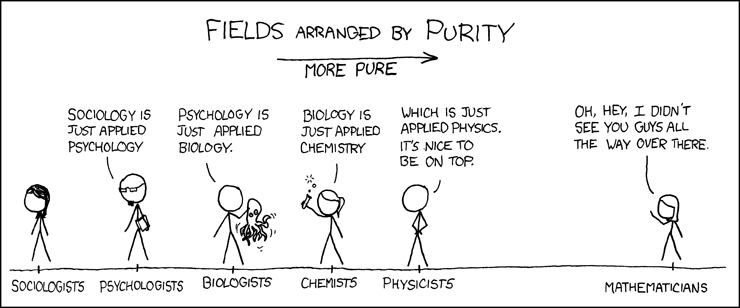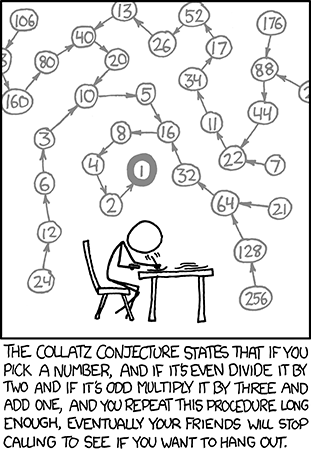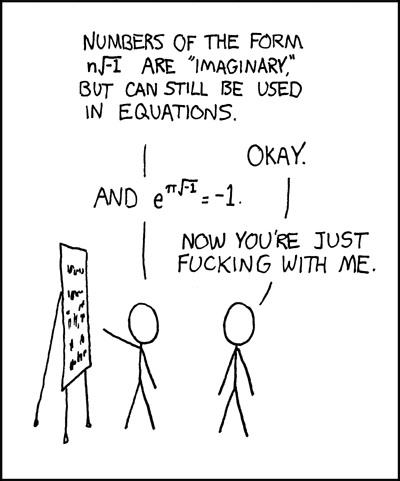You are currently browsing the tag archive for the ‘comics’ tag.http://www.phdcomics.com/comics/archive.php?comicid=27

Definition 1 A vector space${V}$ over the number field${\mathbb{C}}$ or${\mathbb{R}}$ is called an inner product space if it is equipped with an inner product${\left\langle \cdot,\cdot\right\rangle }$ satisfying for all${u,v,w\in V}$ and scalar${c}$,

1.${\left\langle u,u\right\rangle \geq0}$,${\left\langle u,u\right\rangle =0}$ if and only if${u=0}$,
2.${\left\langle u+v,w\right\rangle =\left\langle u,v\right\rangle +\left\langle v,w\right\rangle }$,
3.${\left\langle cu,v\right\rangle =c\left\langle u,v\right\rangle }$, and
4.${\left\langle u,v\right\rangle =\overline{\left\langle v,u\right\rangle }}$.${\mathbb{C}^{n}}$ is an inner product space over${\mathbb{C}}$ with the inner product$\displaystyle \left\langle x,y\right\rangle =y^{*}x=\overline{y_{1}}x_{1}+\cdots+\overline{y_{n}}x_{n}.$

An inner product space over${\mathbb{R}}$ is usually called a Euclidean space.

The following properties of an inner product can be deduced from the four axioms in Definition 1:

1.${\left\langle x,cy\right\rangle =\bar{c}\left\langle x,y\right\rangle }$,
2.${\left\langle x,y+z\right\rangle =\left\langle x,y\right\rangle +\left\langle x,z\right\rangle }$,
3.${\left\langle ax+by,cw+dz\right\rangle =a\bar{c}\left\langle x,w\right\rangle +b\bar{c}\left\langle y,w\right\rangle +a\bar{d}\left\langle x,z\right\rangle +b\bar{d}\left\langle y,z\right\rangle }$,
4.${\left\langle x,y\right\rangle =0}$ for all${y\in V}$ if and only if${x=0}$, and
5.${\left\langle x,\left\langle x,y\right\rangle y\right\rangle =\left|\left\langle x,y\right\rangle \right|^{2}}$.

An important property shared by all inner products is the Cauchy-Schwarz inequality and, for an inner product space, one of the most useful inequalities in mathematics.

Theorem 1 (Cauchy-Schwarz Inequality) Let${V}$ be an inner product space. Then for all vectors${x}$ and${y}$ in${V}$ over the field${\mathbb{C}}$ or${\mathbb{R}}$,$\displaystyle \left|\left\langle x,y\right\rangle \right|^{2}\leq\left\langle x,x\right\rangle \left\langle y,y\right\rangle .$

Equality holds if and only if${x}$ and${y}$ are linearly dependent.

The proof of this can be done in a number of different ways. The most common proof is to consider the quadratic function in${t}$$\displaystyle \left\langle x+ty,x+ty\right\rangle \ge0$

and derive the inequality from the non-positive discriminant. We will first present this proof.

Proof: Let${x,y\in V}$ be given. If${y=0}$, the assertion is trivial, so we may assume that${y\neq0}$. Let${t\in\mathbb{R}}$ and consider$\displaystyle \begin{array}{rcl} p(t) & \equiv & \left\langle x+ty,x+ty\right\rangle \\ & = & \left\langle x,x\right\rangle +t\left\langle y,x\right\rangle +t\left\langle x,y\right\rangle +t^{2}\left\langle y,y\right\rangle \\ & = & \left\langle x,x\right\rangle +2t\, Re\left\langle x,y\right\rangle +t^{2}\left\langle y,y\right\rangle , \end{array}$

which is a real quadratic polynomial with real coefficients. Because of axiom (1.), we know that${p(t)\geq0}$ for all real${t}$, and hence${p(t)}$ can have no real simple roots. The discriminant of${p(t)}$ must therefore be non-positive$\displaystyle (2\, Re\left\langle x,y\right\rangle )^{2}-4\left\langle y,y\right\rangle \left\langle x,x\right\rangle \leq0$

and hence$\displaystyle (Re\left\langle x,y\right\rangle )^{2}\leq\left\langle x,x\right\rangle \left\langle y,y\right\rangle . \ \ \ \ \ (1)$

Since this inequality must hold for any pair of vectors, it must hold if${y}$ is replaced by${\left\langle x,y\right\rangle y}$, so we also have the inequality$\displaystyle (Re\left\langle x,\left\langle x,y\right\rangle y\right\rangle )^{2}\leq\left\langle x,x\right\rangle \left\langle y,y\right\rangle \left|\left\langle x,y\right\rangle \right|^{2}$

But${Re\left\langle x,\left\langle x,y\right\rangle y\right\rangle =Re\overline{\left\langle x,y\right\rangle }\left\langle x,y\right\rangle =Re\left|\left\langle x,y\right\rangle \right|^{2}=\left|\left\langle x,y\right\rangle \right|^{2}}$, so$\displaystyle \left|\left\langle x,y\right\rangle \right|^{4}\leq\left\langle x,x\right\rangle \left\langle y,y\right\rangle \left|\left\langle x,y\right\rangle \right|.^{2} \ \ \ \ \ (2)$

If${\left\langle x,y\right\rangle =0}$, then the statement of the theorem is trivial; if not, then we may divide equation (2) by the quantity${\left|\left\langle x,y\right\rangle \right|^{2}}$ to obtain the desired inequality$\displaystyle \left|\left\langle x,y\right\rangle \right|^{2}\leq\left\langle x,x\right\rangle \left\langle y,y\right\rangle .$

Because of axiom (1.),${p(t)}$ can have a real (double) root only if${x+ty=0}$ for some${t}$. Thus, equality can occur in the discriminant condition in equation (1) if and only if${x}$ and${y}$ are linearly dependent.$\Box$

We will now present a matrix proof, focusing on the complex vector space, which is perhaps the simplest proof of the Cauchy-Schwarz inequality.

Proof: For any vectors${x,y\in\mathbb{C}^{n}}$ we noticed that,$\displaystyle \left[x,y\right]^{*}\left[x,y\right]=\left[\begin{array}{cc} x^{*}x & x^{*}y\\ y^{*}x & y^{*}y \end{array}\right]\geq0.$

By taking the determinant for the${2\times2}$ matrix,$\displaystyle \begin{array}{rcl} \left|\begin{array}{cc} x^{*}x & x^{*}y\\ y^{*}x & y^{*}y \end{array}\right| & = & (x^{*}x)(y^{*}x)-(x^{*}y)(y^{*}x)\\ & = & \left\langle x,x\right\rangle \left\langle x,y\right\rangle -\left\langle y,x\right\rangle \left\langle x,y\right\rangle \\ & = & \left\langle x,x\right\rangle \left\langle x,y\right\rangle -\overline{\left\langle x,y\right\rangle }\left\langle x,y\right\rangle \\ & = & \left\langle x,x\right\rangle \left\langle x,y\right\rangle -\left|\left\langle x,y\right\rangle \right|^{2} \end{array}$

the inequality follows at once,$\displaystyle \left|\left\langle x,y\right\rangle \right|^{2}\leq\left\langle x,x\right\rangle \left\langle y,y\right\rangle .$

Equality occurs if and only if the${n\times2}$ matrix${\left[x,y\right]}$ has rank 1; that is,${x}$ and${y}$ are linearly dependent.$\Box$

References

 Zhang, Fuzhen. Matrix theory: basic results and techniques. Springer Science & Business Media, 2011.Euler Formula

The Euler formula, sometimes also called the Euler identity, states$\displaystyle e^{ix}=cosx+isinx,$

where${i}$ is the imaginary unit. Note that Euler’s polyhedral formula is sometimes also called the Euler formula, as is the Euler curvature formula.

The special case of the formula with${x=\pi}$ gives the beautiful identity$\displaystyle e^{i\pi}=-1,$

an equation connecting the fundamental numbers${i,\pi,e,1,}$ and${0}$, the fundamental operations${+}$,${\times}$, and exponentiation, the most important relation${=}$, and nothing else. Gauss is reported to have commented that if this formula was not immediately obvious, the reader would never be a first-class mathematician.